Click to Chat

1800-1023-196

+91-120-4616500

CART 0

• 0

MY CART (5)

Use Coupon: CART20 and get 20% off on all online Study Material

ITEM
DETAILS
MRP
DISCOUNT
FINAL PRICE
Total Price: Rs.

There are no items in this cart.
Continue Shopping```Algebra of MatricesAny two matrices can be added if they are of the same order and the resulting matrix is of the same order. If two matrices A and B are of the same order, they are said to be conformable for addition.

For example: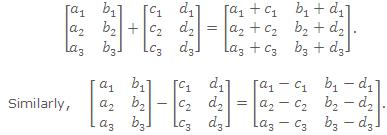Note:

Only matrices of the same order can be added or subtracted.

Addition of matrices is commutative as well as associative.

Cancellation laws hold well in case of addition.

The equation A + X = 0 has a unique solution in the set of all m × n matrices.

Scalar Multiplication

The matrix obtained by multiplying every element of a matrix A by a scalar λ is called the multiple of A by λ and its denoted by λ A i.e. if A = [aij] then λA = [λaij].

For example: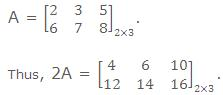Note: All the laws of ordinary algebra hold for the addition or subtraction of matrices and their multiplication by scalar.

Multiplication of Matrices

Two matrices can be multiplied only when the number of columns in the first, called the prefactor, is equal to the number of rows in the second, called the postfactor. Such matrices are said to be conformable for multiplication.where cij = ai1 b1j + ai2 b2j +...+ ain bnj= ∑nk=1  aik bkii = 1, 2, 3 ......, m and
j = 1, 2, 3 ......, p.

Properties of Multiplication

Matrix multiplication may or may not be commutative. i.e., AB may or may not be equal to BA

If AB = BA, then matrices A and B are called Commutative Matrices.
If AB = BA, then matrices A and B are called Anti-Commutative Matrices.

Matrix multiplication is Associative
Matrix multiplication is Distributive over Matrix Addition.
Cancellation Laws not necessary hold in case of matrix multiplication i.e., if AB = AC => B = C even if A ≠ 0.
AB = 0 i.e., Null Matrix, does not necessarily imply that either A or B is a null matrix.

Illustration:

A =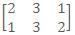and B =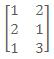, show that AB ≠ BA.

Solution:

Here A.B =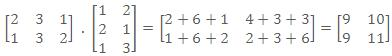and B.A =Thus A.B ≠ B.A.

Illustration:

If Aα =, then find AαAβ.

Solution:

We have.

At askIITians we provide you free study material on Set Theory and Functions and you get all the professional help needed to get through IIT JEE and AIEEE easily. AskIITians also provides live online IIT JEE preparation and coaching where you can attend our live online classes from your home!

To read more, Buy study materials of Matrices and Determinants comprising study notes, revision notes, video lectures, previous year solved questions etc. Also browse for more study materials on Mathematics here.
```### Course Features

• 731 Video Lectures
• Revision Notes
• Previous Year Papers
• Mind Map
• Study Planner
• NCERT Solutions
• Discussion Forum
• Test paper with Video Solution